Courses

# Test: Reduction Of A Simple Distributed Loading

## 14 Questions MCQ Test Engineering Mechanics | Test: Reduction Of A Simple Distributed Loading

Description
This mock test of Test: Reduction Of A Simple Distributed Loading for Mechanical Engineering helps you for every Mechanical Engineering entrance exam. This contains 14 Multiple Choice Questions for Mechanical Engineering Test: Reduction Of A Simple Distributed Loading (mcq) to study with solutions a complete question bank. The solved questions answers in this Test: Reduction Of A Simple Distributed Loading quiz give you a good mix of easy questions and tough questions. Mechanical Engineering students definitely take this Test: Reduction Of A Simple Distributed Loading exercise for a better result in the exam. You can find other Test: Reduction Of A Simple Distributed Loading extra questions, long questions & short questions for Mechanical Engineering on EduRev as well by searching above.
QUESTION: 1

### The uniformly distributed load is having two different values of load per unit length on the ends of the distribution.

Solution:

The answer is no. The uniform distributed load is not having two different values of the load per unit meter on the corner. Rather the distribution is uniform. That is it is having the same value of the same on both of the corners.

QUESTION: 2

### There are two types of loading. The uniformly distributed and the non-uniformly distributed that is the one having two different values at corners.

Solution:

The two forms of the loading are the uniformly distributed and the non-uniformly distributed that is the one having two different values at corners. The uniform distributed load is not having two different values of the load per unit meter on the corner.

QUESTION: 3

### The type of reduction of the loading is different for the uniformly distributed and the non-uniformly.

Solution:

The methods for the reduction of the loadings is different for both uniformly distributed and the non-uniformly. In the latter the simplification uses the quadratic equation. The latter also uses the integration for the same purpose.

QUESTION: 4

Determine the magnitude of the resultant force acting on the shaft shown from left.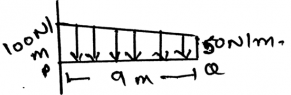Solution:

The net force will act at the centroid of the parabola. Whether it be a parabola or the cubic curve the centroid is the only point at which the net force act. Force can’t be acted horizontally if the loading is vertical. Hence whatever be the shape of the loading, the centroid is the point of action of net force.

QUESTION: 5

In the simplification of the loading system the net force acts at the ___________ of the loading body.

Solution:

In the simplification of the loading system the net force acts at the centroid of the loading body. That is if the loading system is in the form of the triangle then the at the distance 2 by 3 of the base the net force of the loading will act. And the load will be half the area of the loading.

QUESTION: 6

In triangle distributed loading, the loading at any distance can be easily found by using which of the following trigonometry function?

Solution:

In triangle distributed loading, the loading at any distance can be easily found by using Tangent trigonometry function. That is because the linear distance only determines the vertical loading. That is the loading is uniform along the linear distance. Is the linear distance is more the loading is more.

QUESTION: 7

Determine the magnitude of the resultant force acting on the shaft shown. The length is 2m.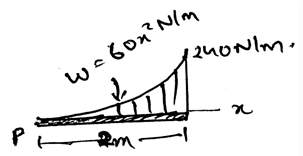Solution:

The net force will act at the centroid of the parabola. Whether it be a parabola or the cubic curve the centroid is the only point at which the net force act. Force can’t be acted horizontally if the loading is vertical. Hence whatever be the shape of the loading, the centroid is the point of action of net force.

QUESTION: 8

If the non-Uniform loading is of the type of parabola then?

Solution:

The net force will act at the centroid of the parabola. Whether it be a parabola or the cubic curve the centroid is the only point at which the net force act. Force can’t be acted horizontally if the loading is vertical. Hence whatever be the shape of the loading, the centroid is the point of action of net force.

QUESTION: 9

If any external force also is applied on the distributed loading then?

Solution:

The external forces are treated differently. They are not added by the force of the distributed loading. That is the force not only acts at the centroid always. It can be shifted also. Depending on the external forces.

QUESTION: 10

The resultant force acting, of the uniformly distributed loading is dependent on:

Solution:

The resultant force acting, of the uniformly distributed loading is dependent on the area that the distribution is covering. The more the area the more is the force. That is the more is the tension created over the structure on which loading is kept. Hence the answer.

QUESTION: 11

The difference between the two types of loading namely uniformly distributed and the non-uniformly distributed loading is that:

Solution:

The non-uniformly distributed loading is using the integration for getting the net force given by it. And the former only need the area or the linear distance of the loading. That is when the distance is multiplied by the uniformly distributed load the net force is obtained. But that is not so with the non-uniform one.

QUESTION: 12

Determine the location of the resultant force acting on the shaft shown from left. The length is 2m.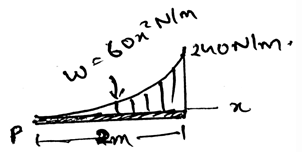Solution:

The net force will act at the centroid of the parabola. Whether it be a parabola or the cubic curve the centroid is the only point at which the net force act. Force can’t be acted horizontally if the loading is vertical. Hence whatever be the shape of the loading, the centroid is the point of action of net force.

QUESTION: 13

Determine the location of the resultant force acting on the shaft shown from left.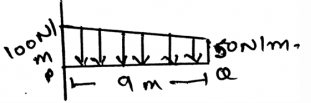Solution:

The net force will act at the centroid of the parabola. Whether it be a parabola or the cubic curve the centroid is the only point at which the net force act. Force can’t be acted horizontally if the loading is vertical. Hence whatever be the shape of the loading, the centroid is the point of action of net force.

QUESTION: 14

Determine the magnitude of the resultant force acting on the shaft shown from left.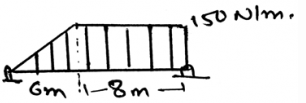Solution:

The net force will act at the centroid of the parabola. Whether it be a parabola or the cubic curve the centroid is the only point at which the net force act. Force can’t be acted horizontally if the loading is vertical. Hence whatever be the shape of the loading, the centroid is the point of action of net force.